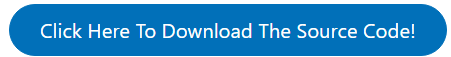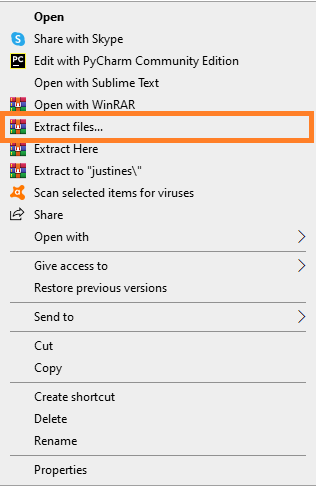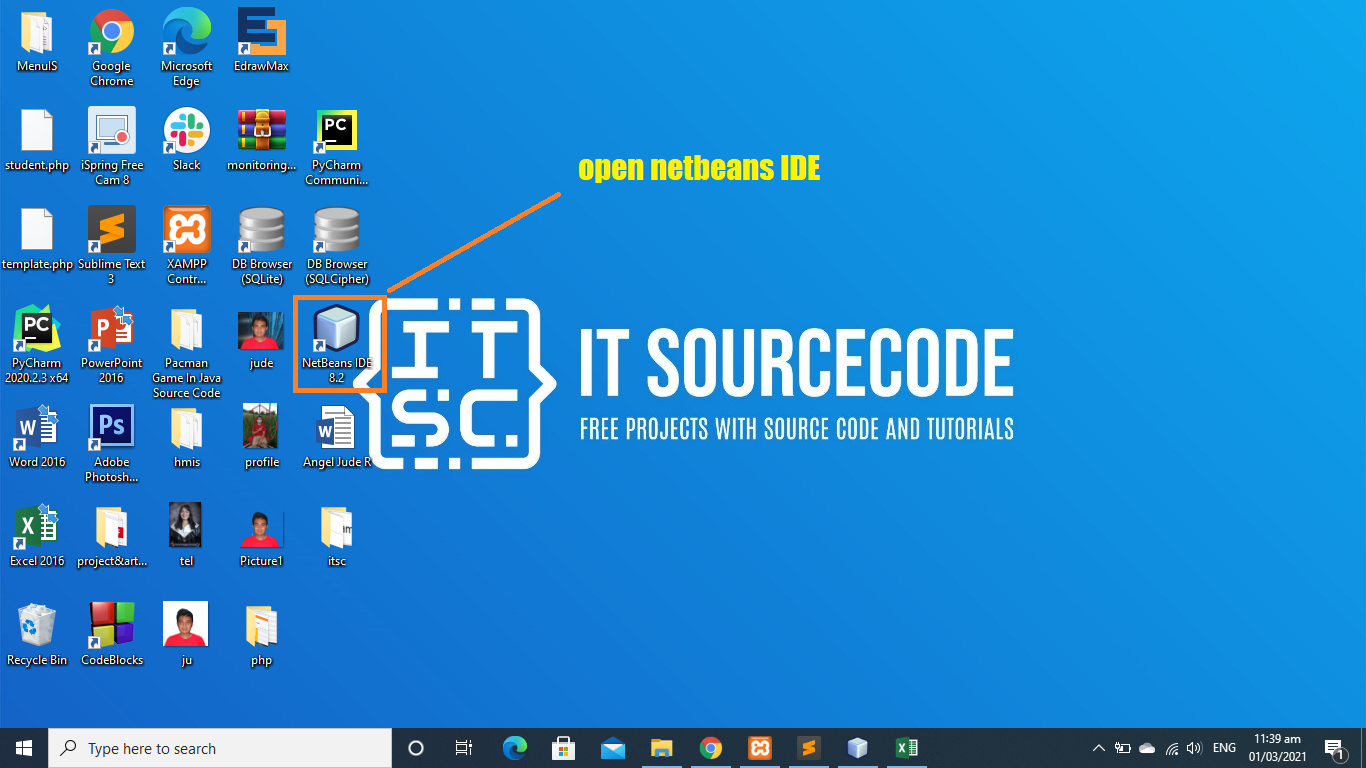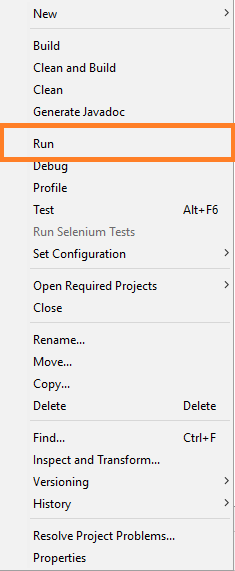The Parking Management System Project In Java Free Download was developed in JAVA Programming using NetBeans IDE, This Java Project With Source Code designed using Graphical User Interface (GUI), This Java Project With Source Code is a sample parking lot system which handles vehicle entry and exit flows.

A Parking Management System Project In Java is used to manage and solve the Parking Problems of the company/Institute. This project is build on Java using NetBeans. The main objective for developing this project is to reduce the traffic in the parking place. It gives information about a big range of parking spaces – small or big.

To start executing a Parking Management System Free Download, makes sure that you have  NeatBeans IDE or any platform of Java installed in your computer.

## Parking Management System Project In Java Free Download : Steps on how to run the project

Time needed: 5 minutes

These are the steps on how to run Parking Management System Project In Java Free Download• Step 2: Extract file.

Second, after you finished download the source code, extract the zip file.• Step 3: Open Netbeans.

Third, open “Netbeans IDE”.• Step 4: Click open project.• Step 5: Run the project.

Fifth, right click the project folder and click run.## The code given below is for the priority class of the project

```/*
* To change this template file, choose Tools | Templates
* and open the template in the editor.
*/
package parking.management;

import java.util.Calendar;
import javax.swing.JOptionPane;

/**
*
*/

class priority {
static String dtm;
static   Calendar cal=Calendar.getInstance();
static  int pi= cal.get(Calendar.HOUR_OF_DAY);
static  int  mi= cal.get(Calendar.MINUTE);

String nw,namw,etimw,dtimw;
static  String []name=new String;
static int []pn=new int;
static String []no=new String;
static String []emailid = new String;
static  String []etime = new String;
static  int []hetime = new int;
static  int []dtime = new int;
static  String []hdtime = new String;
static String []dater = new String;

static   String []payt = new String;
static  int []ammount = new int;
static int front=-1;
static int rear=-1;
public static  int isfull()
{
if(rear==99)
{
JOptionPane.showInputDialog(null,"Warning","full");
return 1;
}
else
{
return 0;
}
}

/**
*
* @return
*/
public static int isempty()
{
if(front==-1)
{
JOptionPane.showInputDialog(null,"Warning","empty");
return 1;

}
else
{
return 0;

}
}

public  static void enqueue(String nam,String n,String emaili ,
String etim,int dtim, String pay, int ammoun,String date,int p)
{
System.out.print(dtim);
int i=0;
int j=0;
if(front==-1 && rear==-1){
front=0;
rear=0;
name[rear]=nam;
no[rear]=n;
emailid[rear]=emaili;
etime[rear]=etim;
dtime[rear]=dtim;
payt[rear]=pay;
ammount[rear]=ammoun;
dater[rear]=date;
pn[rear]=p;
rear++;
return;
}
if(isfull()==1)
{
return;
}
for(i=front;i<rear;i++)
{

if(dtime[i]>dtim)
{

for(j=rear-1;j>=0;j--)
{
name[j+1]=name[j];
no[j+1]=no[j];
emailid[j+1]=emailid[j];
etime[j+1]=etime[j];
dtime[j+1]=dtime[j];
payt[j+1]=payt[j];
ammount[j+1]=ammount[j];
dater[j+1]=dater[j];
pn[j+1]=pn[j];

}
name[j+1]=nam;
no[j+1]=n;
emailid[j+1]=emaili;
etime[j+1]=etim;
dtime[j+1]=dtim;
payt[j+1]=pay;
ammount[j+1]=ammoun;
dater[j+1]=date;
pn[j+1]=p;
rear++;
return;
}
}
name[rear]=nam;
no[rear]=n;
emailid[rear]=emaili;
etime[rear]=etim;
dtime[rear]=dtim;
payt[rear]=pay;
ammount[rear]=ammoun;
dater[j+1]=date;
pn[j+1]=p;

rear++;
}

public static int peekd(){

return  dtime[front];

}

static String peekc() {
return name[front];
}
static String peeke() {
return emailid[front];
}

static void deque(){
if(front==-1){
System.out.println("queue is empty");
return ;}
else{

le.insert(name[front],no[front], emailid[front], etime[front], dtime[front], payt[front], ammount[front],dater[front],pn[front]);
front++;
System.out.println("element removed");

}
if(front==rear){

front=-1;
rear=-1;
}

return ;

}
public static void deletespecific(int p)
{
int i=front;

while(pn[i]!=p)
{
i++;

}
dtm=pi+":"+mi;

viewlink.insert(name[i],no[i], emailid[i], etime[i], dtm, payt[i], ammount[i],dater[i],pn[i]);
for(int j=i;j<rear-1;j++)
{
name[j]=name[j+1];
no[j]=no[j+1];
emailid[j]=emailid[j+1];
etime[j]=etime[j+1];
dtime[j]=dtime[j+1];
payt[j]=payt[j+1];
ammount[j]=ammount[j+1];
dater[j]=dater[j+1];
pn[j]=pn[j+1];

}

rear--;

}
public  String[] find(int p)
{

int i=Math.max(front, 0);
System.out.println(pn.length+"plength"+" i="+i);
while(pn[i]!=p && i<rear)
{
i++;

}
if(i>rear)
{
String []s={"1"};

return s;
}
if(i==rear&& pn[i]!=p)
{
String []s={"1"};
return s;
}
if(pn[i]==p&&i==rear)
{nw= no[i];
System.out.print(nw);
namw=     name[i];
etimw=  etime[i];
dtimw= dtime[i]/60+ ":"+dtime[i]%60;
System.out.print(namw);
System.out.print(etimw);
System.out.print(dtimw);
String []s={namw,etimw,dtimw,nw};
return s;
}
nw= no[i];
System.out.print(nw);
namw=     name[i];
etimw=  etime[i];
dtimw= dtime[i]/60+ ":"+dtime[i]%60;
System.out.print(namw);
System.out.print(etimw);
System.out.print(dtimw);
String []s={namw,etimw,dtimw,nw};
return s;

}

}

```

In this module which is the main class of the system project.

## About The Parking Management System In Java

Anyway, if you want to level up your programming knowledge, especially Java, try this new article I’ve made for you Best Java Projects With Source Code For Beginners Free Download 2021.

## Summary

The Java Project With Source Code is built fully in Java and MySQL Database. It has full-featured Graphical User Interface (GUI) with all the functionalities

This Article is the way to enhance and develop our skills and logic ideas which is important in practicing the Java programming language which is most well known and most usable programming language in many company.

## Inquiries

### 7 thoughts on “Parking Management System Project In Java Free Download”

1.2.3.4.what is the password for the zip file?

•5.•